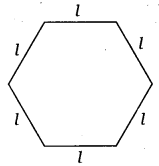# Class 6 Maths NCERT Solutions for Chapter 11 Algebra Ex – 11.2

## Algebra

Question 1.
The side of an equilateral triangle is shown by l. Express the perimeter of the equilateral triangle using l.

Solution:
If P represents the perimeter of an equilateral triangle of side l, then P = l + l + l= 3 x l

Question 2.
The side of a regular hexagon (see figure) is denoted by l. Express the perimeter of the hexagon using l.Solution:
If P represents the perimeter of a regular hexagon of side l, then P = l + l + l + l + l + l = 6 x l.

Question 3.
A cube is a three-dimensional figure as shown in figure. It has six faces and all of them are identical squares. The length of an edge of the cube is given by l. Find the formula for the total length of the edges of a cube.Solution:
Since all the 12 edges of a cube are of same length, l. Then, their total length = 12 x l.

Question 4.
The diameter of a circle is a line, which joins two points on the circle and also passes through the centre of the circle. (In the adjoining figure AB is a diameter of the circle;

C is its centre). Express the diameter of the circle (d) in terms of its radius (r).Solution:
Diameter AB = AC + CB
If d represents the diameter of the circle and r is the radius of circle, then
d = CP + CP [∵ CP = r]
d = r + r = 2r

Question 5.
To find sum of three numbers 14, 27 and 13, we can have two ways.

(a) We may first add 14 and 27 to get 41 and then add 13 to it to get the total sum 54 or
(b) We may add 27 and 13 to get 40 and then add 14 to get the sum 54.
Thus, (14 + 27) + 13 = 14 + (27 + 13).
This can be done for any three numbers. This property is known as the associativity of addition of numbers. Express this property which we have already studied in the chapter on Whole Numbers, in a general way, by using variables a, b and c.

Solution:
The given properly is stated as
For 3 variables a, b and c, we have
(a + b) + c = a + (b + c)

### Take Your IIT JEE Coaching to Next Level with Examtube

• Mentoring & Teaching by IITians
• Regular Testing & Analysis
• Preparation for Various Engineering Entrance Exams
• Support for School/Board Exams